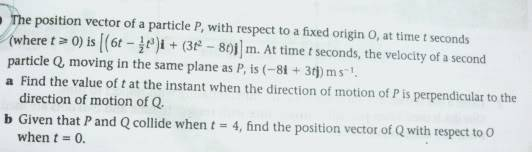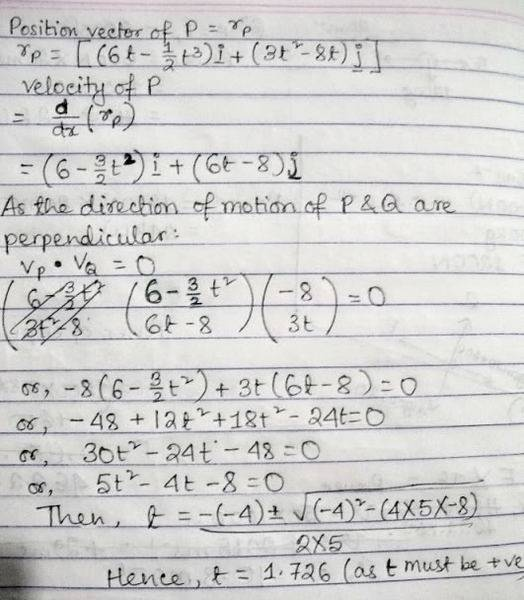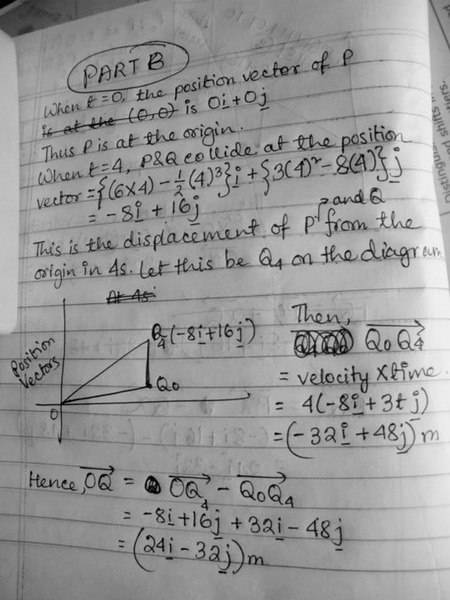A Level Mechanics: Kinematics of 2 particles moving in the same plane [i, j vectors]

Hello :)
I'm self-studying for my Edexcel Mechanics 2 GCE A Level exam (it's in 10 days :S) and was having lots of trouble with one of the kinematics questions. I've uploaded photos of the problem and my solution for part (a) of the question. I couldn't use the math notation feature on the Physics Forum properly; I hope the photos aren't a problem for anyone!

Homework StatementHomework Equations

* When a and b are two perpendicular vectors, a.b = 0.

The Attempt at a Solution

My working out for part (a) of the question:My answer (t = 1.726s) doesn't match with the book's answer of t = 2s. As the book wants the 'instant' when the direction of motion of P is perpendicular to that of Q, I don't think that the book wants me to round off 1.726 to 2.

I'm completely clueless about part (b), so I would really appreciate it if someone could kindly provide some hints to get me in the right direction. I could try solving it then. If you need any further information, please ask and I'll try to provide it. I'm just very, very stumped with this question and have nobody who I can ask. :( Some fresh ideas might really help!

I'm getting the exact same answer, yours seems spot on.

Unless we're crappy at taking derivatives, the given answer is just wrong.

$$\vec V_P (t=2 sec) = 4\hat y$$

$$\vec V_Q (t=2 sec) = -8 \hat x + 6 \hat y$$

And clearly these two are not perpendicular.

Have you taken a swing at part B yet? Do you know what to do?

Hi RoyalCat :) Thank-you for your help. I'm guessing my book is wrong, unless someone else shows us otherwise? :) I've finally come up with something for part B. My answer of (24i - 32j)m for the position vector of Q with respect to O when t=0 doesn't match the book's answer of (24i - 8j)m.

Here's my working out:Is my book wrong here as well? This is my examining body's (Edexcel) new and revised official coursebook for the Mechanics 2 exam :S :S

The book is right on this one, though.

The velocity of Q is not constant. It is a function of time.
The difference in position must be found by integration.
One approach is to take the indefinite integral, and set two constants for the initial x and y positions, which must then be found using the boundary conditions (The position at time t=4 sec is known).

Thank-you so much for your hints RoyalCat :) My answer finally matches the book's one:

Position vector of Q
= Integrating the velocity of Q
= (-8t + a)i + [(3/2)t^2 + b]j

As P and Q collide when t = 4, the position vector of Q = position vector of P at that time

Hence, position vector of Q when t = 4: -8i + 16j {worked this out in my first attempt at part B, above}

So,
-8t + a = -8
So, a = 24

And, (3/2)t^2 + b = 16
So, b = -8

Hence, position vector of Q = (-8t + 24)i + [(3/2)t^2 - 8]j

Therefore, when t = 0
Position vector of Q = 24i - 8j (The answer ^_^)

Spot on. :) Well done!